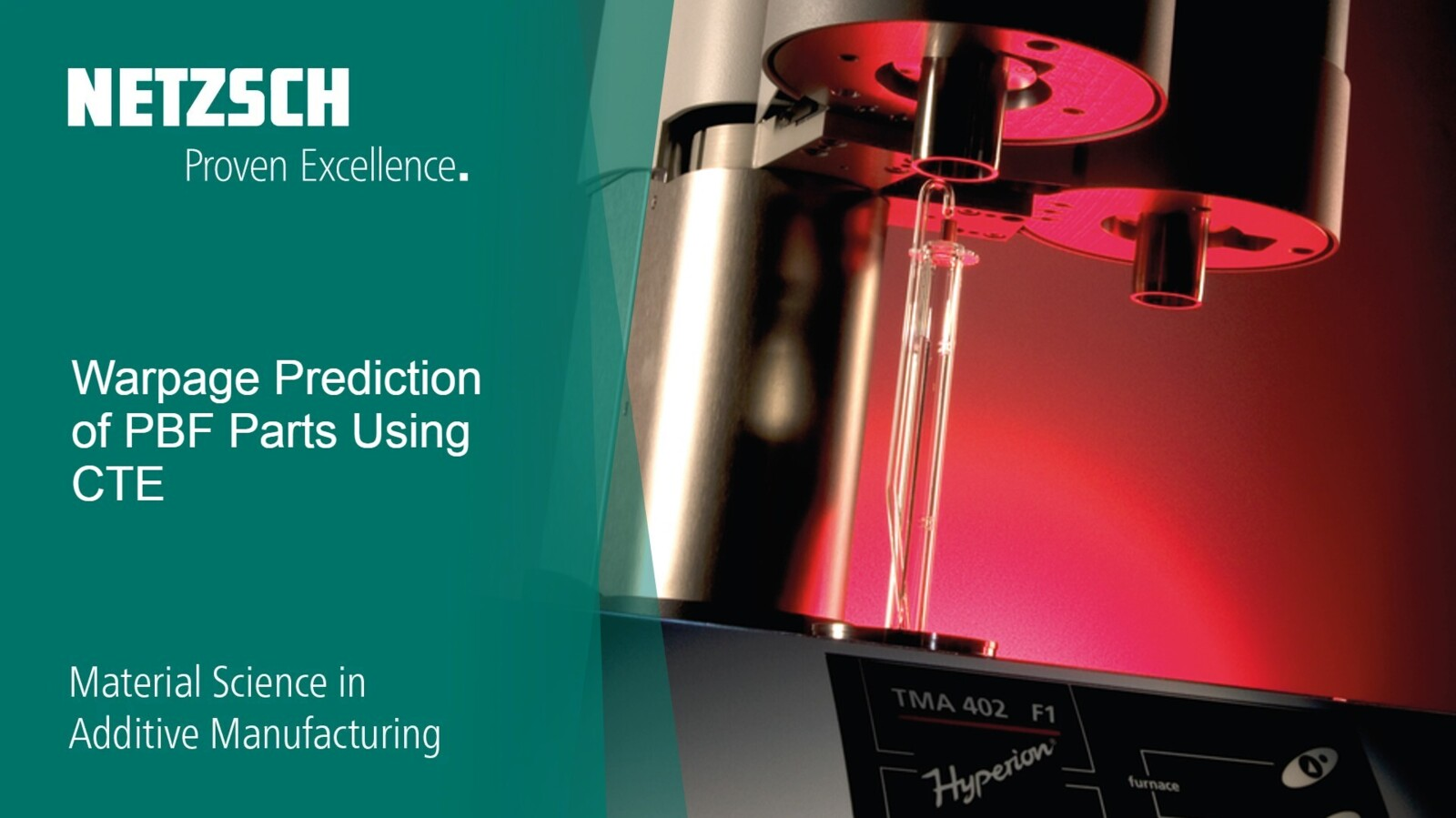06.10.2021 by Aileen Sammler, Dr. Natalie Rudolph

# Material Science in Additive Manufacturing: Warpage Prediction of PBF Parts Using CTE

Residual StressStress is defined as a level of force applied on a sample with a well-defined cross section. (Stress = force/area). Samples having a circular or rectangular cross section can be compressed or stretched. Elastic materials like rubber can be stretched up to 5 to 10 times their original length.stress can lead to warpage of a 3D-printed part. Both Dynamic Mechanical and Thermomechanical Analysis are capable to estimate warpage. In this video, Dr. Natalie Rudolph explains, how the coefficient of thermal expansion (Coefficient of Linear Thermal Expansion (CLTE/CTE)The coefficient of linear thermal expansion (CLTE) describes the length change of a material as a function of the temperature.CTE), measured with Thermomechanical Analysis, can be used to predict and calculate warpage.

In our last episodes, we focused mainly on thermal material behavior measured with Differential Scanning Calorimetry (DSC). Today, Dr. Nathalie Rudolph will talk In our last video, we talked about how residual StressStress is defined as a level of force applied on a sample with a well-defined cross section. (Stress = force/area). Samples having a circular or rectangular cross section can be compressed or stretched. Elastic materials like rubber can be stretched up to 5 to 10 times their original length.stress can lead to warpage of a 3D-printed part and how Dynamic Mechanical Analysis (DMA) can help estimate residual stress. Today, Dr. Natalie Rudolph explains how warpage can be predicted using the coefficient of thermal expansion (CTE) with the help of Thermomechanical Analysis (TMA).

#### This week’s topic: Warpage Prediction of PBF Parts Using CTE

We use Thermomechanical Analysis (TMA) to measure the deformation of a substance under a non-oscillatory load as a function of temperature and time while the substance is subjected to a controlled temperature program in a specific atmosphere.

• How to estimate and calculate the coefficient of thermal expansion CTE using the thermomechanical analysis method
• Using the information of thermomechanical analysis to predict warpage of a part printed with PA12 powder
• The curling effect on Powder Bed Fusion